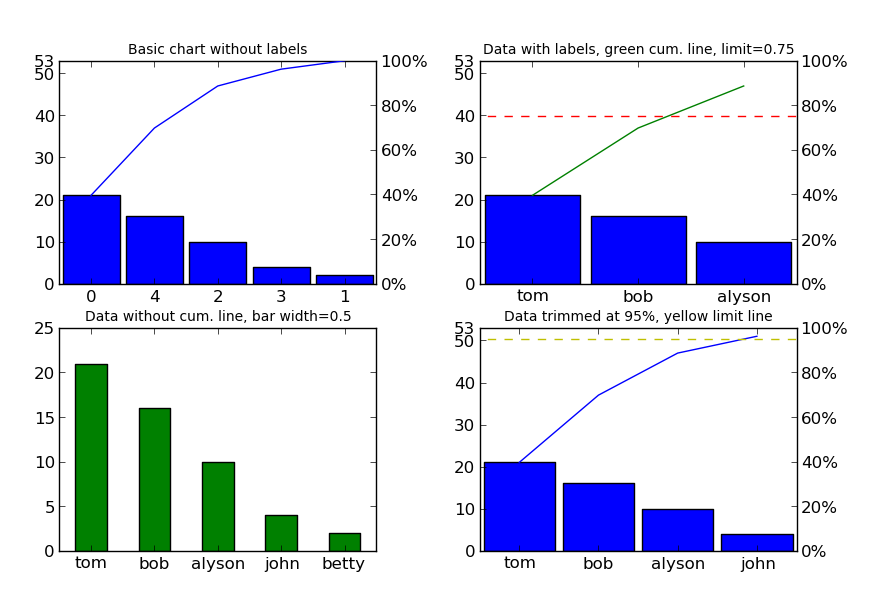Pareto chart for python (similar to Matlab's, but much more flexible) - Fork from @tintrinh

## Project description

Pareto chart for python 3 (similar to Matlab, but much more flexible) - Fork from @tintrinh.

## Features

• Data labels for the chart x-axis.
• Fully customizable with unique arg and kwarg inputs:
• Bar chart: follows the inputs of the matplotlib.pyplot.bar function (use bar_args=(...) and bar_kw={...}).
• Cumulative line: follows the inputs of the matplotlib.pyplot.plot function (use line_args=(...) and line_kw={...}).
• Limit line: follows the inputs of the matplotlib.axes.Axes.axhline function (use limit_kw={...}).
• Put the chart on arbitrary axes.

## Examples

First, a simple import::

from paretochart.paretochart import pareto


Now, let's create the numeric data (no pre-sorting necessary)::

data = [21, 2, 10, 4, 16]


We can even assign x-axis labels (in the same order as the data)::

labels = ['tom', 'betty', 'alyson', 'john', 'bob']


For this example, we'll create 4 plots that show the customization capabilities::

import matplotlib.pyplot as plt

# create a grid of subplots
fig, axes = plt.subplots(2, 2)


The first plot will be the simplest usage, with just the data::

pareto(data, axes=axes[0, 0])
plt.title('Basic chart without labels', fontsize=10)


In the second plot, we'll add labels, put a cumulative limit at 0.75 (or 75%) and turn the cumulative line green::

pareto(data, labels, axes=axes[0, 1], limit=0.75, line_args=('g',))
plt.title('Data with labels, green cum. line, limit=0.75', fontsize=10)


In the third plot, we'll remove the cumulative line and limit line, make the bars green and resize them to a width of 0.5::

pareto(data, labels, cumplot=False, axes=axes[1, 0], data_kw={'width': 0.5,
'color': 'g'})
plt.title('Data without cum. line, green bar width=0.5', fontsize=10)


In the fourth plot, let's put the cumulative limit at 95% and make that line yellow::

pareto(data, labels, limit=0.95, axes=axes[1, 1], limit_kw={'color': 'y'})
plt.title('Data trimmed at 95%, yellow limit line', fontsize=10)


And last, but not least, let's show the image::

fig.canvas.set_window_title('Pareto Plot Test Figure')
plt.show()


This should result in the following image (click here if the image doesn't show up):## Installation

Since this is really a single python file, you can simply go to the GitHub_ page, simply download paretochart.py and put it in a directory that python can find it.

Alternatively, the file can be installed using::

pip install --upgrade rogeriopradoj-paretochart


If you are using Python2, you can use original @tintrinh's project::

pip install --upgrade paretochart


## Development and Publishing New Versions

• define next version number (try to follow semver 2
• make the changes in codebase
• update setup.py:
• version
• update README.md:
• pareto_plot_test_figure url
• commit, tag, and push to git central repo
• create a source distribution, and validate it:
• python setup.py sdist
• twine check dist/*
• upload the source to PyPi:
• twine upload dist/*

## Project details

This version2.0.0

Uploaded source